# Android OpenGLES2.0（十八）——轻松搞定Blend颜色混合

Blend是OpenGL中的一个非常重要的部分，它可以让每个输出的源和目的颜色以多种方式组合在一起，以呈现出不同的效果，满足不同的需求。

## Blend相关函数及意义

//调用此方法，传入GL_BLEND开启BLEND功能
void glEnable(GLenum cap);
//调用此方法，出入GL_BLEND关闭BLEND功能
void glDisable(GLenum cap);
//设置BLEND颜色，结合glBlendFuncSeparate或glBlendFunc使用
void glBlendColor(GLclampf red,GLclampf green,GLclampf blue,GLclampf alpha);
//设置BLEND方程式
void glBlendEquation(GLenum mode);
//对RGB和Alpha分别设置BLEND方程式
void glBlendEquationSeparate(GLenum modeRGB,GLenum modeAlpha);
//设置BLEND函数
void glBlendFunc(GLenum sfactor,GLenum dfactor);
//对RGB和Alpha分别设置BLEND函数
void glBlendFuncSeparate(GLenum srcRGB,GLenum dstRGB,GLenum srcAlpha,GLenum dstAlpha);

Blend的使用比较简单，但是如果不理解Blend的这些函数及参数的意义，使用了错误的参数，就难以获得我们所期望的混合结果了。

glBlendFuncglBlendFuncSeparate都是设置混合因子，反正就是这么个意思了。区别在于glBlendFunc是设置RGBA的混合因子，而glBlendFuncSeparate是分别设置RGB和Alpha的混合因子。设置混合因子是做什么的呢？继续看。
glBlendEquationglBlendEquationSeparate都是设置Blend的方程式，也就是设置混合的计算方式了，具体参数后面说。他们的区别在同glBlendFuncglBlendFuncSeparate的区别一样。

## glBlendFunc及glBlendFuncSeparate详细说明

glBlendFuncSeparate设置混合因子，参数及它们表示的主要如下，而glBlendFunc的参数也是这些，表示的意义就是RGB和A合并为RGBA就是了。在下表中，s0表示源，d表示目的，c表示有glBlendColor设置进来的常量。

Parameter RGB Factor Alpha Factor
GL_ZERO (0, 0, 0) 0
GL_ONE (1, 1, 1) 1
GL_SRC_COLOR (Rs0, Gs0, Bs0) As0
GL_ONE_MINUS_SRC_COLOR (1, 1, 1) - (Rs0, Gs0, Bs0) 1 - As0
GL_ONE_MINUS_DST_COLOR (1, 1, 1) - (Rd, Gd, Bd) 1 - Ad
GL_SRC_ALPHA (As0, As0, As0) As0
GL_ONE_MINUS_SRC_ALPHA (1, 1, 1) - (As0, As0, As0) 1 - As0
GL_CONSTANT_COLOR (Rc, Gc, Bc) Ac
GL_ONE_MINUS_CONSTANT_COLOR (1, 1, 1) - (Rc, Gc, Bc) 1 - Ac
GL_CONSTANT_ALPHA (Ac, Ac, Ac) Ac
GL_ONE_MINUS_CONSTANT_ALPHA (1, 1, 1) - (Ac, Ac, Ac) 1 - Ac
GL_SRC_ALPHA_SATURATE (i, i, i) 1

## glBlendEquation及glBlendEquationSeparate详细说明

glBlendEquationSeparate的设置混合操作，参数及其意义如下表所示。通过glBlendEquationSeparate或者glBlendEquation设置的方程中，源和目的颜色分别为(Rs,Gs,Bs,As)$(R_s, G_s, B_s, A_s)$(Rd,Gd,Bd,Ad)$(R_d, G_d, B_d, A_d)$。最终混合的颜色结果为(Rr,Gr,Br,Ar)$(R_r, G_r, B_r, A_r)$。源和目的的混合因子分别为(sR,sG,sB,sA)$(s_R, s_G, s_B, s_A)$(dR,dG,dB,dA)$(d_R, d_G, d_B, d_A)$。其中，所有的颜色分量的取值范围都为[ 0, 1 ]。GL_MIN和GL_MAX是在OpenGLES3.0才有的

Mode RGB Components Alpha Component
GL_FUNC_ADD RrGrBr=sRRs+dRRd=sGGs+dGGd=sBBs+dBBd\begin{align}R_r & = s_R R_s + d_R R_d\\G_r & = s_G G_s + d_G G_d\\B_r & = s_B B_s + d_B B_d\end{align} Ar=sAAs+dAAd$A_r = s_A A_s + d_A A_d$
GL_FUNC_SUBTRACT RrGrBr=sRRsdRRd=sGGsdGGd=sBBsdBBd\begin{align}R_r & = s_R R_s - d_R R_d\\G_r & = s_G G_s - d_G G_d\\B_r & = s_B B_s - d_B B_d\end{align} Ar=sAAsdAAd$A_r = s_A A_s - d_A A_d$
GL_FUNC_REVERSE_SUBTRACT RrGrBr=dRRdsRRs=dGGdsGGs=dBBdsBBs\begin{align}R_r & = d_R R_d - s_R R_s\\G_r & = d_G G_d - s_G G_s\\B_r & = d_B B_d - s_B B_s\end{align} Ar=dAAdsAAs$A_r = d_A A_d - s_A A_s$
GL_MIN RrGrBr=min(Rs,Rd)=min(Gs,Gd)=min(Bs,Bd)\begin{align}R_r & = min(R_s, R_d)\\G_r & = min(G_s, G_d)\\B_r & = min(B_s, B_d)\end{align} Ar=min(As,Ad)$A_r = min(A_s, A_d)$
GL_MAX RrGrBr=max(Rs,Rd)=max(Gs,Gd)=max(Bs,Bd)\begin{align}R_r & = max(R_s, R_d)\\G_r & = max(G_s, G_d)\\B_r & = max(B_s, B_d)\end{align} Ar=max(As,Ad)$A_r = max(A_s, A_d)$

## Blend代码示例

@Override
public void onSurfaceCreated(GL10 gl, EGLConfig config) {
GLES20.glClearColor(0,0,0,0);
mSrcFilter.create();
mDstFilter.create();
int[] textures=new int;

//导入一张图片设置为源纹理
GLES20.glGenTextures(2,textures,0);
GLES20.glBindTexture(GLES20.GL_TEXTURE_2D,textures);
EasyGlUtils.useTexParameter();
GLUtils.texImage2D(GLES20.GL_TEXTURE_2D,0,GLES20.GL_RGBA,srcBitmap,0);
mSrcFilter.setTextureId(textures);
//再导入一张图片设置为目标纹理
GLES20.glBindTexture(GLES20.GL_TEXTURE_2D,textures);
EasyGlUtils.useTexParameter();
GLUtils.texImage2D(GLES20.GL_TEXTURE_2D,0,GLES20.GL_RGBA,dstBitmap,0);
mDstFilter.setTextureId(textures);

}

@Override
public void onSurfaceChanged(GL10 gl, int width, int height) {
this.width=width;
this.height=height;
mSrcFilter.setSize(width,height);
mDstFilter.setSize(width,height);
MatrixUtils.getMatrix(mDstFilter.getMatrix(),MatrixUtils.TYPE_FITSTART,
dstBitmap.getWidth(),dstBitmap.getHeight(),width,height);
MatrixUtils.getMatrix(mSrcFilter.getMatrix(),MatrixUtils.TYPE_FITSTART,
srcBitmap.getWidth(),srcBitmap.getHeight(),width,height);
}

@Override
public void onDrawFrame(GL10 gl) {
GLES20.glClear(GLES20.GL_COLOR_BUFFER_BIT | GLES20.GL_DEPTH_BUFFER_BIT);
//开启Blend
GLES20.glEnable(GLES20.GL_BLEND);
//设置BlendFunc，第一个参数为源混合因子，第二个参数为目的混合因子
GLES20.glBlendFunc(nSrcPar,nDstPar);
//设置BlendEquation，GLES2.0中有三种
GLES20.glBlendEquation(equaInt[nEquaIndex]);
GLES20.glViewport(0,0,width,height);
//先渲染目的纹理出来，再渲染源纹理出来，是源纹理去与目的纹理混合
mDstFilter.draw();
mSrcFilter.draw();
}1. 当目标和源因子都设置为GL_ZERO，无论混合方程怎样设置，最终肯定啥也没有。
2. 当源设置为GL_ONE，目标设置为GL_ZERO，方程设置为加还是减，最终应该渲染的就是目标的颜色，也就是之渲染出金币。
3. 当源设置为GL_ONE，目标设置为GL_SRC_COLOR，方程设置为加，根据公式最终颜色=(目标颜色*目标因子)+(源颜色*源因子)，得到最终有颜色的区域必定是源alpha不为0的区域，因为源是作为目标因子的，源*目标，最终源中alpha为0的区域，这个结果也为0，也就是最终的结果区域透明了。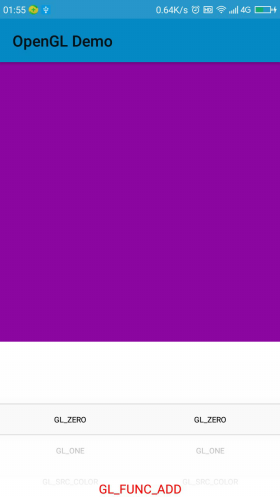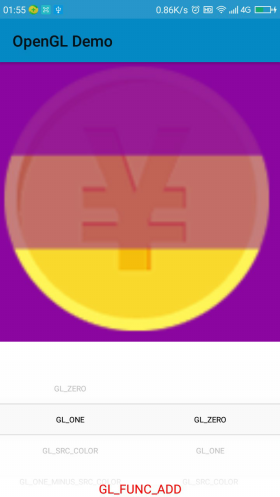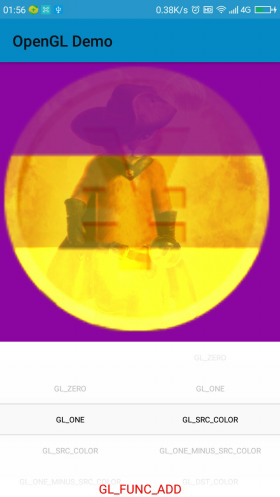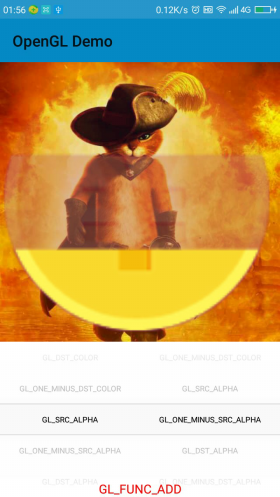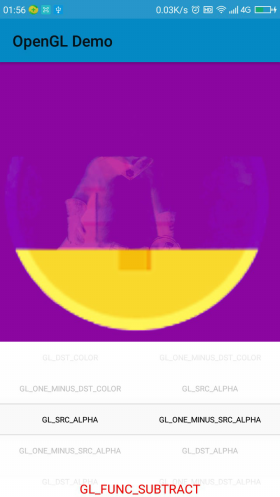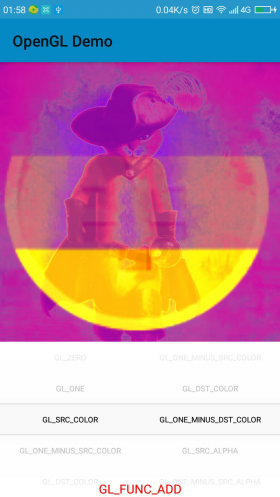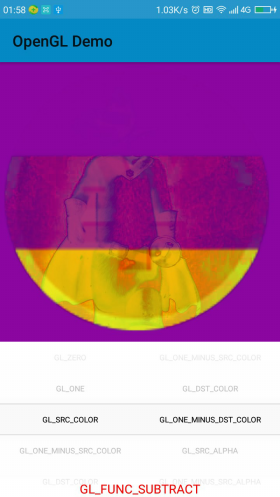## 源码

02-218016

#### 《高效学习OpenGL》 之 混合 glBlendFunc(), glBlendFuncSeparate(),glBlendEquation(), glBlendEquationSeparate()03-19261

#### OpenGL中的Blend

08-161108

#### Opengl-混合(原来不理解的src dst blender总算理解了)

11-28658

#### GLES2.0中文API-glBlendColor

06-122万+

#### OpenGL中的混合 (blend)

11-16575

#### Opengl es2.0 学习笔记（九）颜色混合

10-121万+

#### Android OpenGLES2.0（一）——了解OpenGLES2.0

03-234319

#### 自定义View基础：Android中的颜色和颜色混合©️2020 CSDN 皮肤主题: 大白 设计师: CSDN官方博客点击重新获取扫码支付1.余额是钱包充值的虚拟货币，按照1:1的比例进行支付金额的抵扣。
2.余额无法直接购买下载，可以购买VIP、C币套餐、付费专栏及课程。余额充值